# Heat transfer

We placed a lead object weighing 0.4 kg and 250°C in 0.4 L water. What was the initial temperature of water t2 if the object's temperature and the water after reaching equilibrium was 35°C? We assume that the heat exchange occurred only between the lead object and water. The mass heat capacity of lead is 130 J/kg / °C. The mass heat capacity of water is 4200 J/kg / °C.

t2 =  28.3452 °C

### Step-by-step explanation: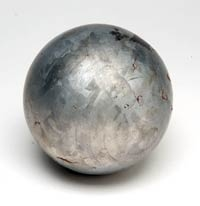Did you find an error or inaccuracy? Feel free to write us. Thank you!Tips to related online calculators
Do you know the volume and unit volume, and want to convert volume units?
Tip: Our Density units converter will help you with the conversion of density units.
Do you want to convert mass units?

## Related math problems and questions:

• The aluminumThe aluminum object with a weight of 0.80 kg and a temperature of 250°C was placed in the water with a weight of 1.6 kg and a temperature of 23°C. What is the system temperature after reaching equilibrium? We assume that the heat exchange occurred only be
• The bathThe bath has water with a volume of 220 l and a temperature of 65°C. Determine the mass m2 of water at 15°C to be poured so that the resulting temperature of the water in the bath is 40°C. We neglect the heat exchange between water and the environment. T
• Ice to boiling water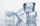Calculate the heat needed to convert 5kg of ice with a temperature of -5°C to water with a temperature of 100°C. c (water) = 4200 J. Kg-1. kg-1, c (ice) = 2100 J. Kg-1. kg-1. the mass group heat of solidification of water is l = 334kJ/kg. Calculate the he
• Latent heat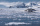How much heat is needed to take from 100 g of water at 20°C to cool to ice at -18°C? Mass heat capacity c (ice) = 21kJ/kg / °C; c (water) = 4.19 KJ/kg / °C and the mass group heat of solidification of water is l = 334kJ/kg
• Thermometer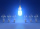Immerse a thermometer with a heat capacity of 2.0 J. K-1 in water weighing 67.0 grams. Before immersion in water, the thermometer showed a temperature of 17.8 degrees Celsius. After reaching equilibrium, the temperature is 32.4 degrees. What was the water
• Receiving heatHow many degrees Celsius was an iron cylinder weighing 300 g heated in a water bath if it received heat of 7.2 kJ? (specific heat capacity of iron c = 0.46kJ/kg / ºC)
• Electric cooker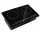During which time t does an electric cooker with power input P = 500 W and with efficiency n = 75% heat water with mass m = 2 kg and temperature t1 = 10°C to the boiling point (t2 = 100°C). The specific heat capacity of water is c = 4 180 J. Kg-1. K-1
• Basic formExpressed the ratios of values in the basic form: 0,5 t : 1,2 kg 200 l : 0,15 m3 12 t : 3600 kg 500 kg : 2,5 t 0,9 kg : 500 g 3,6 m : 240 cm 1200 mm : 2,4 m 300 l : 0,3 m3 6 min 30 s : 900 s
• ThereThere is 5 l of water with a temperature of 20°C in the bucket. We add 3 liters of hot water. The resulting temperature will be 30°C. What was the temperature of the hot water?
• Water temperaturesTo 25 liters of water at 50 °C we add 15 liters of water at a different temperature. How many °C should the water be colder than 50 °C so that 40 liters of the water obtained has a temperature 42.5 °C?
• Cuboid 5Calculate the mass of the cuboid with dimensions of 12 cm; 0.8 dm and 100 mm made from spruce wood (density = 550 kg/m3).
• Dynamometer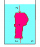What is the volume of a body that stretches a dynamometer in air, on which it is suspended by a force of 2.5 N, and if it is immersed in alcohol with a density of 800 kg/m ^ 3, does it tension the dynamometer with a force of 1.3 N?
• Swing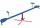A child weighing 12 kg is sitting on a swing at a distance of 130 cm from the rotation axis. How far away from the rotation (center) axis must sit down his mother weighs 57 kg if she wants to be a swing in balance?
• Above water surface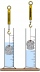If we remove the stone from the water, we apply a force of 120N. How much force will we have to exert if we move the stone above the water surface? The density of the stone is 5000 kg/m ^ 3.
• Copper wireWhat is the weight in kg of copper wire 200 m long with a diameter of 0.6 cm if the density of copper is 8.8 kg?
• Water mixingWe have 520 ml of hot water and 640 ml of water at 48°C. What is the temperature of approximately hot water when the resulting mixture has a temperature of 65°C?
• Potatoes bags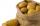I have three bags with 21 kg potatoes. The first bag is 5.5 kg more than in the second bag, and the third is 0.5 kg more than in the second bag. Determine how many kgs of potatoes are in each bag.